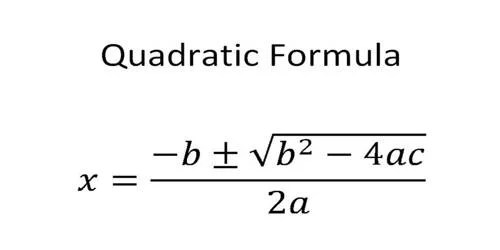Education

# Quadratic Equations and Formula Explain

Any equation that can be rearranged in the standard form ax2 + bx + c = 0 is known as a quadratic equation. There are many methods that can be used to solve a quadratic equation, such as completing the squares, factoring, or using the quadratic formula.

In this article, we will take a detailed look at what is a quadratic equation and how to solve problems based on the same.

## What is a Quadratic Equation?

Polynomial equations that are of degree 2 and have only one type of variable are known as quadratic equations. Such equations are univariate as they have only one unknown.

As mentioned above, the standard representation for such an equation is ax2 + bx + c = 0, where a, b are coefficients, x is the variable, and c is the constant. A quadratic equation always has two roots.

### How to Solve Quadratic Equations?

A quadratic equation has two solutions irrespective of whether the coefficients are real or complex. These are called roots and can be real or imaginary as well as distinct or not distinct.

### Factoring Method

We can express a quadratic equation as a product of the factors. This is usually the first method that kids are introduced to when learning this topic. Given below are the steps that have to be followed in order to break down the problem and find its solution.

• Step 1: We take all the terms on one side of the equation (say L.H.S) and keep zero on the other side (R.H.S).
• Step 2: Next, we factor the equation.
• Step 3: There will be two factors. We equate each factor to 0.
• Step 4: We solve each equation and get the value of the unknown.
• Step 5: We can verify if our answer is correct by plugging the value of the variable in the original equation. On solving, it should yield 0.

### Completing the Squares

The following steps have to be followed while using this method to solve quadratic equations.

• Divide the entire equation with the coefficient a. (from the standard form)
• Keep the variable terms on one side of the equation while the constant term on the other side.
• Add the square of ½ of the current coefficient of x to both sides.
• The left side is written as a square and the right side is simplified.
• Take the square root on both sides.
• To get the value of the unknown, solve these equations.

You can find the roots of a quadratic equation by applying the following formula.

x = [-b ± √(b² -4ac)]/2a

where the notations are from the quadratic equation in the standard form.

The nature of the roots can be determined by the discriminant give by

D = b2 – 4ac.

### Nature of Roots

• There are two real roots if the discriminant is positive.
• There is exactly one real root if the discriminant is zero.
• There are two distinct complex roots if the discriminant is negative.Conclusion

Quadratic Equations form a very important part of mathematics and see wide industrial as well as academic applications. The best way to learn such a complicated topic is by taking the services of an institution such as Cuemath.

The CueMath is one such online educational platform that provide children the opportunity to avail the best quality of education at the right price. The math tutors use many fun and interactive resources that help kids build a strong conceptual foundation in the subject. Thus, a kid is sure to master a topic like quadratic equations in no time!